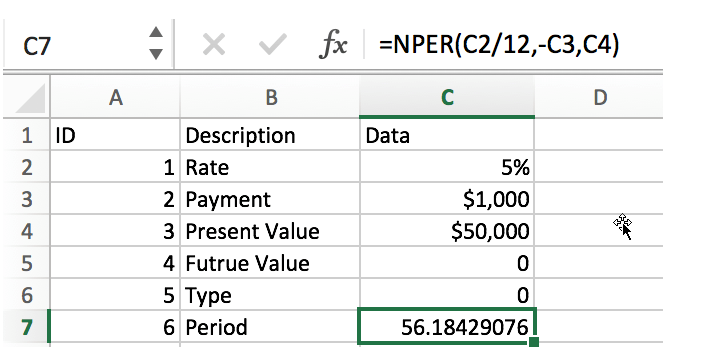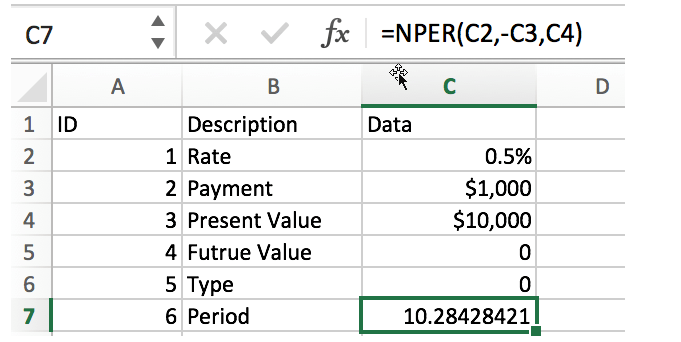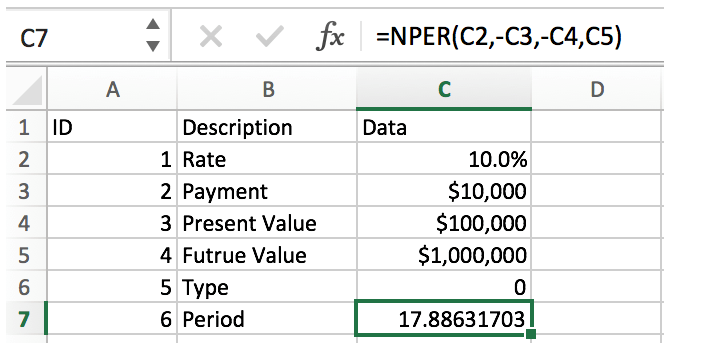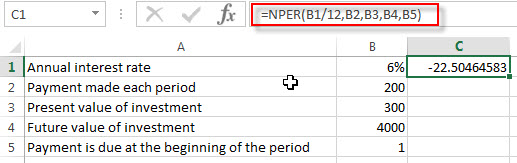## Calculate The Period of Loan or Investment in Excel

In our daily life, most of us will invest or take a loan, so we need to master some simple financial functions to calculate the period needed for loans or investments. If you work as an accountant, you need to master some basic Excel financial functions even more.

In fact, Excel provides many basic financial functions (such as IRR, FV, PMT, etc.). By applying these functions, we can make a simple estimate of interest rate, period, payment, etc.

Today we will introduce an Excel financial function NPER. The function name consists of Number + Period. It is a function often used by accountants. In addition to the basic introduction of the function, we will also provide some examples to illustrate the usage of the function.

## NPER FUNCTION

Excel NPER function is a financial function, based on a fixed interest rate and equal payments, used to calculate the number of periods of a loan or investment.

### Syntax:

`=NPER(rate,pmt,pv,[fv],[type])`

### Arguments:

• rate – it is the interest rate for each period, which is a fixed value
• pmt – it is the amount payable for each period and remains constant throughout the annuity period. Typically, payment includes principal and interest, but excludes other fees and taxes.
• pv – present value. This is the sum of the amounts already accounted for from the beginning of the calculation of the investment, or the cumulative sum of the current values of future payments, also known as the principal.
• fv – future value [optional]. The cash balance after the last payment, if it is omitted, is assumed to have a value of zero, i.e., the future value of a loan is zero.
• type – 0 or 1 to specify whether the payment for each period is at the beginning or at the end of the period. Optional.

## NPER FUNCTION EXAMPLES

### Example1: Calculate the number of repayment periods for the loan

To repay a loan of 50,000 with an annual interest rate of 5% and a monthly repayment of 1,000 (at the end of each month), calculate the number of months required to repay the loan.In this example, the formula is:

`=NPER(C2/12,-C3,C4)`

Rate:

C2/12 (5%/12). Rate in C2 is annual interest rate. Since we want to know the number of periods (months) to repay the loan, we use C2/12 to get the monthly interest rate.

PMT:

-C3. The equal payment is \$1000. Because it is used to pay the loan, it is a cash outflow, so it has a negative value. So for the argument payment, it has a value of “-C3”.

PV:

C4, present value is \$50000. That means we will pay for a loan of \$50000.

FV:

The future value of the loan is 0.

Type:

Type is 0. At the end of period.

In this example, the result is 56.18, so it takes 57 months to repay the loan.

### Example2: Calculate the number of installments

Suppose an item is purchased at a cost of 10,000, and the monthly payment of 1,000 is made at the end of each month, and the monthly compounding rate is 0.5%, calculate the number of months of repayment.In this example, the formula is:

`=NPER(C2,-C3,C4)`

This example is similar to the previous one. The difference is that this is a monthly interest rate, so you don’t have to divide it by 12 months.

### Example3: Calculate the cumulative investment period

Suppose the annual rate of return on investment is 10%, now you have invested \$100000, add \$10000 every year, calculate the number of years to reach 1 million.In this example, the formula is:

`=NPER(C2,-C3,-C4,C5)`

In this example, we are calculating the number of years it would take to reach \$1 million with a 10% annual return, so the period is also “years”. Since payments and present value are paid for investments, they are cash outflows, so they are negative in the formula. The future value is out expected value \$1 million.

### Related Functions

• Excel NPER Function
The Excel NPER function returns the number of periods for an investment or loan based on periodic payment amount and a constant interest rate.The NPER function is a build-in function in Microsoft Excel and it is categorized as a Financial Function.…

## Excel NPER Function

This post will guide you how to use Excel NPER function with syntax and examples in Microsoft excel.

### Description

The Excel NPER function returns the number of periods for an investment or loan based on periodic payment amount and a constant interest rate.

The NPER function is a build-in function in Microsoft Excel and it is categorized as a Financial Function.

The NPER function is available in Excel 2016, Excel 2013, Excel 2010, Excel 2007, Excel 2011 for Mac.

### Syntax

The syntax of the NPER function is as below:

`= NPER (rate, pmt, pv, [fv], [type])`

Where the NPER function arguments are:

• Rate -This is a required argument. The interest rate per period.
• Pmt– This is a required argument. The amount of the payment made each period. And if the Pmt argument is omitted, it will set as the default value 0.
• Pv – This is an optional argument. The present value of the payments. And if the PV argument is omitted, it will be set the default value as 0.
• FV– This is an optional argument. The Future value of the loan/investment at the end of nper payments. If it is omitted, it will be set the default value as 0.
• Type – This is an optional argument. It indicates when the payments are due. And if the type argument is omitted, it will be set as 0.  And the Type argument can have two value 0 or 1.
 Set type equal to If payments are due 0 At the end of the period 1 At the beginning of the period

### Excel NPER Function Examples

The below examples will show you how to use Excel NPER Function to get the number of payment periods for a loan.

#1 to get the periods for the investment, using the following formula:

`=NPER(B1/12,B2,B3,B4,B5)`### Related Functions

• Excel FV Function
The Excel FV function used to calculate the future value of an investment based on a constant interest rate. The syntax of the FV function is as below:=FV(rate,nper,pmt,[pv],[type])…
• Excel IPMT Function
The Excel IPMT function used to calculate the interest payment for an investment based on a constant payment schedule and a constant interest rage.The syntax of the IPMT function is as below:= IPMT (rate, per, nper, pv, [fv], [type])…
• Excel IRR Function
The Excel IRR function returns the internal rate of return for a series of cash flows and the cash flows must be occurred at regular intervals (monthly or annually).The syntax of the IRR function is as below:=IRR(values, [guess])…
• Excel ISPMT Function
The Excel ISPMT function used to calculate the interest paid during a specific period of an investment.The syntax of the ISPMT function is as below:= ISPMT (rate, per, nper, pv)…
• Excel MIRR Function
The Excel MIRR function returns the modified internal rate of return for a series of cash flows and the cash flows must be occurred at regular intervals (monthly or annually). The syntax of the MIRR function is as below:=MIRR(values, finance_rate,reinvest_rate)…
• Excel NPV Function
The Excel NPV function returns the net present value of an investment by using a discount rate and a series of future cash flows (future payments and income).The syntax of the NPV function is as below:= NPV (rate, Value1,[value2],…)…
• Excel PMT Function
The Excel PMT function returns the payment amount for a loan or investment based on constant payments and a constant interest rate.The syntax of the PMT function is as below:= PMT(rate, nper, pv,[fv],[type])…
• Excel PPMT Function
The Excel PPMT function returns the payment amount on the principal for a given period for a loan or investment based on constant payments and a constant interest rate. The syntax of the PPMT function is as below:=PPMT(rate, per,nper, pv,[fv],[type])…
• Excel RATE Function
The Excel RATE function returns the interest rate per payment period of an annuity.The syntax of the RATE function is as below:=RATE(nper, pmt,pv,[fv],[type],[guess])…
• Excel PV Function
The Excel PV function returns the present value of a loan or investment based on constant payments and a constant interest rate. So you can use the PV function to get the present value based on a series of future payments.The syntax of the PV function is as below:= PV(rate,nper,pmt,[fv],[type])…